Gromacs  2020.1
gmx::ExponentialMovingAverage Class Reference

#include <gromacs/math/exponentialmovingaverage.h>

## Description

Evaluate the exponential moving average with bias correction.

The exponential moving average at the 0th data point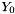is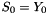and at the n-th data point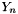with n>0 it is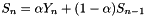, where the smoothing factor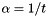is determined via a time constant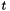.

To avoid large impact of the first data point in a "burn-in" phase, the weight of points are unbiased by substituting for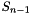above,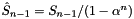.

## Public Member Functions

ExponentialMovingAverage (real timeConstant, const ExponentialMovingAverageState &state={})
Construct by setting the time constant and state. Allows reinitiating with data from memory. More...

void updateWithDataPoint (real dataPoint)
Update the moving average with a data point.

real biasCorrectedAverage () const
The exponential weighted average with bias correction.

bool increasing () const
Returns true if last added data point increased the average.

const
ExponentialMovingAverageState
state () const
Return the current state of the exponential moving average.

real inverseTimeConstant () const
The inverse time constant for the exponential moving average.

## Constructor & Destructor Documentation

 gmx::ExponentialMovingAverage::ExponentialMovingAverage ( real timeConstant, const ExponentialMovingAverageState & state = {} )

Construct by setting the time constant and state. Allows reinitiating with data from memory.

Parameters
 [in] timeConstant time in number of data points [in] state of the exponential moving average
Exceptions
 InconsistentInputError if timeConstant < 1

The documentation for this class was generated from the following files: